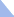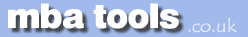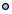MarketingFinanceOperations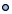EconomicsStatisticsStrategyMeetingsToolboxCommunicationmbatools.co.ukcompiled by

gari jenkins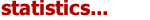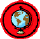Without knowing something about how data is dispersed, measures of central tendency may be misleading. For example, a residential street with 20 homes on it having a mean value of £200,000 with little variation from the mean would be very different from a street with the same mean home value but with 3 homes having a value of £1 million and the other 17 clustered around £60,000. Measures of dispersion provide a more complete picture. Dispersion measures include the range, average deviation, variance, and standard deviation.

Range

The simplest measure of dispersion is the range. The range is calculated by simply taking the difference between the maximum and minimum values in the data set. However, the range only provides information about the maximum and minimum values and does not say anything about the values in between.

Average Deviation

Another method is to calculate the average difference between each data point and the mean value, and divide by the number of points to calculate the average deviation (mean deviation). However, performing this calculation will result in an average deviation of zero since the values above the mean will cancel the values below the mean. If this method is used, the absolute value of the difference is taken so that only positive values are obtained, and the result sometimes is called the mean absolute deviation. The average deviation is not very difficult to calculate, and it is intuitively appealing. However, the mathematics are very complex when using it in subsequent statistical analysis. Because of this complexity, the average deviation is not a very commonly used measure of dispersion.

Variance and Standard Deviation

A better way to measure dispersion is to square the differences before averaging them. This measure of dispersion is known as the variance, and the square root of the variance is known as the standard deviation. The standard deviation and variance are widely used measures of dispersion.Statistics Revision Sheets